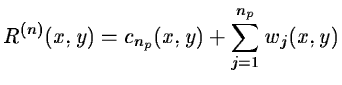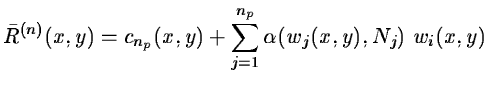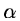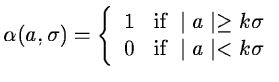Next: Regularization of Van Cittert's Up: Deconvolution Previous: Tikhonov's regularization and multiresolution

## Regularization from significant structures

If we use an iterative deconvolution algorithm, such as Van Cittert's or Lucy's one, we define R(n)(x,y), the error at iteration n:
 R(n)(x,y) = I(x,y) - P(x,y) * O(n)(x,y) (14.111)

By using the à trous wavelet transform algorithm, R(n) can be defined by the sum of its np wavelet planes and the last smooth plane (see equation 14.33).(14.112)

The wavelet coefficients provide a mechanism to extract from the residuals at each iteration only the significant structures. A large part of these residuals are generally statistically non significant. The significant residual is:(14.113)

Nj is the standard deviation of the noise at scale j, andis a function which is defined by:(14.114)

The standard deviation of the noise Nj is estimated from the standard deviation of the noise in the image. This is done from the study of noise variation in the wavelet space, with the hypothesis of a white Gaussian noise.

We now show how the iterative deconvolution algorithms can be modified in order to take into account only the significant structure at each scale.Next: Regularization of Van Cittert's Up: Deconvolution Previous: Tikhonov's regularization and multiresolution
Petra Nass
1999-06-15